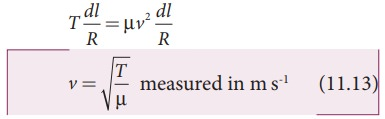Home | | Physics 11th std | Velocity of transverse waves in a stretched string

# Velocity of transverse waves in a stretched string

Let us compute the velocity of transverse travelling waves on a string.

we shall derive the velocity of waves in two different cases:

1. The velocity of a transverse waves along a stretched string.

2. The velocity of a longitudinal waves in an elastic medium.

Velocity of transverse waves in a stretched string

Let us compute the velocity of transverse travelling waves on a string. When a jerk is given at one end (left end) of the rope, the wave pulses move towards right end with a velocity v as shown in the Figure 11.15 (a). This means that the pulses move with a velocity v with respect to an observer who is at rest frame. Suppose an observer also moves with same velocity v in the direction of motion of the wave pulse, then that observer will notice that the wave pulse is stationary and the rope is moving with pulse with the same velocity v.Consider an elemental segment in the string as shown in the Figure 11.15 (b). Let A and B be two points on the string at an instant of time. Let dl and dm be the length and mass of the elemental string, respectively. By definition, linear mass density, μ isThe elemental string AB has a curvature which looks like an arc of a circle with centre at O, radius R and the arc subtending an angle θ at the origin O as shown in Figure 11.15(b). The angle θ can be written in terms of arc length and radius as θ = dl/R. The centripetal acceleration supplied by the tension in the string isThen, centripetal force can be obtained when mass of the string (dm) is included in equation (11.7)The centripetal force experienced by elemental string can be calculated by substituting equation (11.6) in equation (11.8) we getThe tension T acts along the tangent of the elemental segment of the string at A and B. Since the arc length is very small, variation in the tension force can be ignored. We can resolve T into horizontal component Tcos(θ/2) and vertical component T sin(θ/2).

The horizontal components at A and B are equal in magnitude but opposite in direction; therefore, they cancel each other. Since the elemental arc length AB is taken to be very small, the vertical components at A and B appears to acts vertical towards the centre of the arc and hence, they add up. The net radial force Fr isSince the amplitude of the wave is very small when it is compared with the length of the string, the sine of small angle is approximated as sin(θ/2)≈ θ/2. Hence, equation (11.10) can be written asBut θ =  dl/R, therefore substituting in equation (11.11), we getApplying Newton’s second law to the       elemental string in the radial direction, under equilibrium, the radial component of the force is equal to the centripetal force. Hence equating equation (11.9) and equation (11.12), we have### Observations:

The velocity of the string is

a. directly proportional to the square root of the tension force

b. inversely proportional to the square root of linear mass density

c. independent of shape of the waves.

### EXAMPLE 11.6

Calculate the velocity of the travelling pulse as shown in the figure below. The linear mass density of pulse is 0.25 kg m-1. Further, compute the time taken by the travelling pulse to cover a distance of 30 cm on the string.### Solution

The tension in the string is T = m g = 1.2 × 9.8 = 11.76 N

The mass per unit length is μ = 0.25 kg m-1. Therefore, velocity of the wave pulse isThe time taken by the pulse to cover the distance of 30 cm iswhere

ms = milli second.

Study Material, Lecturing Notes, Assignment, Reference, Wiki description explanation, brief detail
11th Physics : UNIT 11 : Waves : Velocity of transverse waves in a stretched string |